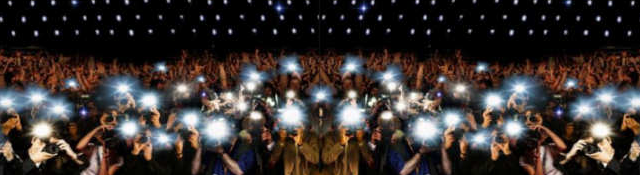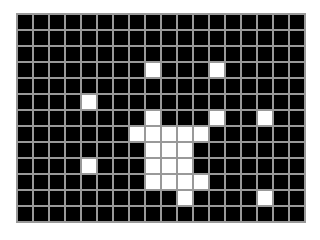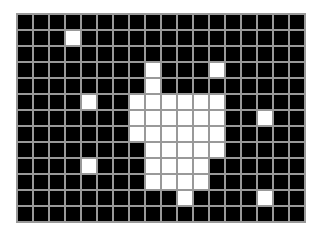## Image Moments

An Image moment is a number calculated using a certain formula. Understand what that formula means might be hard at first. In fact, I got a lot of questions about moments from the tracking tutorial I did long back. So, here it is - an explanation of what moments area!

## The math of moments

In pure math, the nth order moment about the point c is defined as:This definition holds for a function that has just one independent variable. We're interested in images - they have two dimensions. So we need two independent variables. So the formula becomes:Here, the f(x, y) is the actual image and is assumed to be continuous. For our purposes, we need a discrete way (think pixels) to describe moments:The intergrals has been replaced by summations. The order of the moment is m + n. Usually, we calculate the moments about (0, 0). So you can simply ignore the constants cx and cy.

Now with the math part out of the way, let's have a look at what you can calculate with this thing.A binary image with white and black pixels

## Calculating area

To calculate the area of a binary image, you need to calculate its zeroth moment:The x0 and y0 don't have any effect and can be removed.Now, in a binary image, a pixel is either 0 or 1. So for every white pixel, a '1' is added to the moment - effectively calculating the area of the binary image! Another thing to note is that there is only one zeroth order moment.

## Centroid

To calculate the centroid of a binary image you need to calculate two coordinates -How did I get that? Here's a quick explanation. Consider the first moment:The two summations are like a for loop. The x coordinate of all white pixels (where f(x, y) = 1) is added up.

Similarly, we can calculate the sum of y coordinates of all white pixels:Now we have the sum of several pixels' x and y coordinates. To get the average, you need to divide each by the number of pixels. The number of pixels is the area of the image - the zeroth moment. So you get:andOne interesting thing about this technique is that it is not very sensitive to noise. The centroid might move a little bit but not much.

Also, from the math it's clear this technique holds only for single blobs. If you have two white blobs in your image, the centroid will be somewhere in between. You'll have to extract each blob separately to get their centroids.Calculating the centroid of this blob

## Central moments

In fact, this kind of division is very common - dividing a moment by the zeroth order moment. It's so common that it has a name of its own - central moments.

So to calculate the centroid, you need to calculate the first order central moments.

## Higher order moments

Going onto higher order moments, things get complicated really fast. You have  three 2nd order moments, four 3rd order moments, etc. You can combine several of these  moments so that they are translation invariant, scale invariant and even rotation invariant.

While reading about moments, I found an entire book dedicated to pattern recognition with moments. In fact, there are terms called skewness and kurtosis. These refer to third and fourth order moments. They measure how skewed an image is and whether an image is tall and thin or short and fat. Clearly, there's a LOT that can be learned about these mathematical tools.

Utkarsh Sinha created AI Shack in 2010 and has since been working on computer vision and related fields. He is currently at Microsoft working on computer vision.